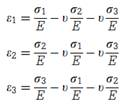### Create an Account

Home / Questions / Show that the elastic stress strain relations for an isotropic material are Hence derive...

# Show that the elastic stress strain relations for an isotropic material are Hence derive an equation for the dilatation in terms of Poissons ratio Youngs modulus and the sum of the principal

Show that the elastic stress–strain relations for an isotropic material areHence derive an equation for the dilatation in terms of Poisson’s ratio, Young’s modulus, and the sum of the principal stresses. For what value of n is the volume change zero?

Jun 26 2020 View more View LessSubscribe To Get Solution